>
>搜索“JPE

1 / 3
• 大图
• 列表

78

• 商品信息
• 品牌 型号 适用标准 类别
价格
预计出货日
• 购买数量
• JPE
• AAL-Y3(SET)
• 通用
• 多管路快速接头
• 询价
• 5个工作日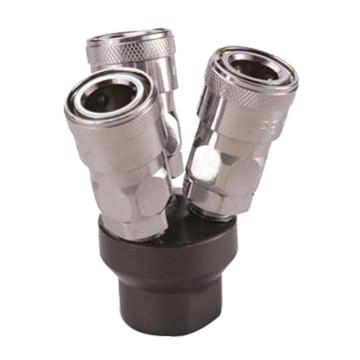- +
• JPE
• AAL-Y2(SET)
• 通用
• 多管路快速接头
• 询价
• 5个工作日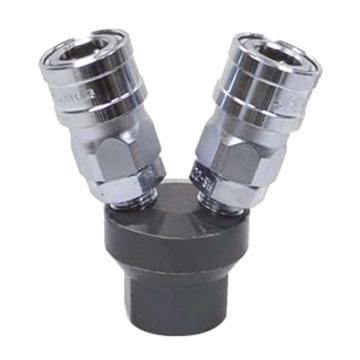- +
• JPE
• AFE-48PH
• 通用
• 胶管插头
• 询价
• 5个工作日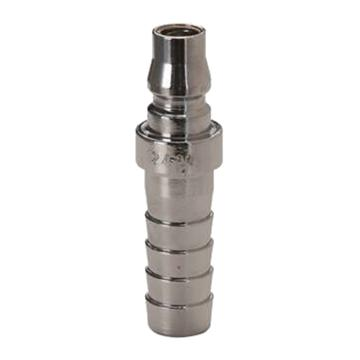- +
• JPE
• AFE-48SH
• 通用
• 胶管插座
• 询价
• 5个工作日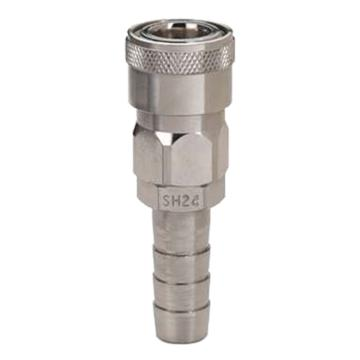- +
• JPE
• AFE-48PM-R
• 通用
• 外牙插头
• 询价
• 5个工作日- +
• JPE
• AFE-48PF-R
• 通用
• 内牙插头
• 询价
• 5个工作日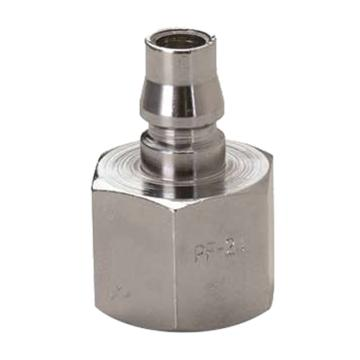- +
• JPE
• AFE-48SM-R
• 通用
• 外牙插座
• 询价
• 5个工作日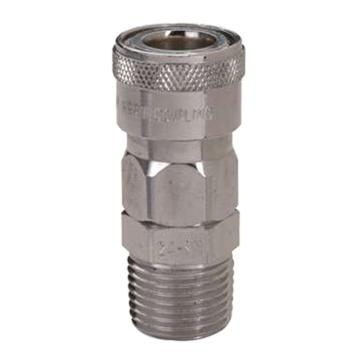- +
• JPE
• AFE-48SF-R
• 通用
• 内牙插座
• 询价
• 5个工作日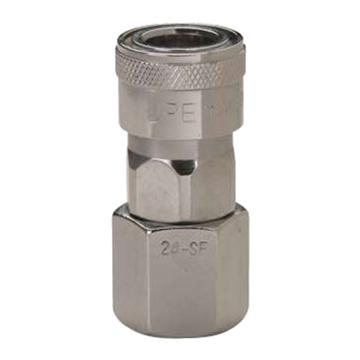- +
• JPE
• AFE-24PH
• 通用
• 胶管插头
• 询价
• 有库存- +
• JPE
• AFE-22SH
• 通用
• 胶管插座
• 询价
• 有库存- +
• JPE
• AFE-44PH
• 通用
• 胶管插头
• 询价
• 有库存- +
• JPE
• AFE-23SH
• 通用
• 胶管插座
• 询价
• 有库存- +
• JPE
• AFE-23PH
• 通用
• 胶管插头
• 询价
• 5个工作日- +
• JPE
• AFE-21PH
• 通用
• 胶管插头
• 询价
• 5个工作日- +
• JPE
• AFE-22PH
• 通用
• 胶管插头
• 询价
• 5个工作日- +
• JPE
• AFE-44SH
• 通用
• 胶管插座
• 询价
• 5个工作日- +
• JPE
• AFE-46SH
• 通用
• 胶管插座
• 询价
• 5个工作日- +
• JPE
• AFE-46PH
• 通用
• 胶管插头
• 询价
• 5个工作日- +
• JPE
• AFE-24SH
• 通用
• 胶管插座
• 询价
• 5个工作日- +
• JPE
• AFE-21SH
• 通用
• 胶管插座
• 询价
• 5个工作日- +
• JPE
• AFE-24PM-R
• 通用
• 外牙插头
• 询价
• 有库存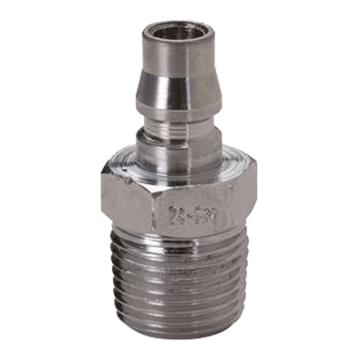- +
• JPE
• AFE-21PM-R
• 通用
• 外牙插头
• 询价
• 有库存- +
• JPE
• AFE-24SM-R
• 通用
• 外牙插座
• 询价
• 有库存- +
• JPE
• AFE-22SF-R
• 通用
• 内牙插座
• 询价
• 有库存- +
• JPE
• AFE-23PM-R
• 通用
• 外牙插头
• 询价
• 有库存- +
• JPE
• AFE-22PF-R
• 通用
• 内牙插头
• 询价
• 有库存- +
• JPE
• AFE-22PM-N
• 通用
• 外牙插头
• 询价
• 有库存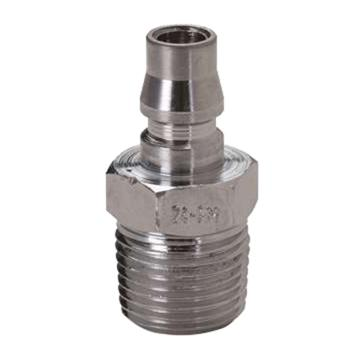- +
• JPE
• AFE-23PM-N
• 通用
• 外牙插头
• 询价
• 有库存- +
• JPE
• AFE-22SM-R
• 通用
• 外牙插座
• 询价
• 有库存- +
• JPE
• AFE-22PM-R
• 通用
• 外牙插头
• 询价
• 有库存- +
• JPE
• AFE-44PM-R
• 通用
• 外牙插头
• 询价
• 5个工作日- +
• JPE
• AFE-21PF-R
• 通用
• 内牙插头
• 询价
• 5个工作日- +
• JPE
• AFE-23PF-R
• 通用
• 内牙插头
• 询价
• 5个工作日- +
• JPE
• AFE-46PM-R
• 通用
• 外牙插头
• 询价
• 5个工作日- +
• JPE
• AFE-24PF-R
• 通用
• 内牙插头
• 询价
• 5个工作日- +
• JPE
• AFE-44PF-R
• 通用
• 内牙插头
• 询价
• 5个工作日- +